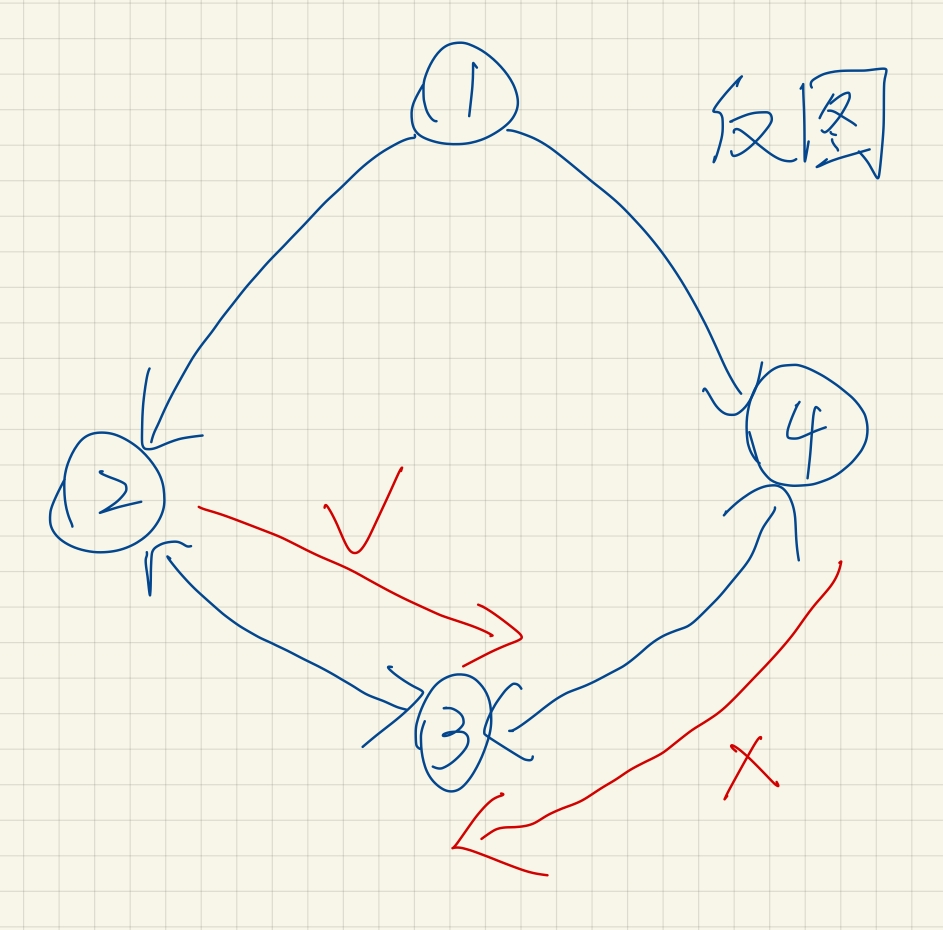# 题目描述

## Solution

T 直接指向的点的集合为 D ，设 Q \in D\forall I \in DI \neq Q

（有个小技巧是：从 Q 能走到哪个点（不包括 Q 自己），那么这个点就一定不是答案）（当 2 走向 3 后，3 被标记，4 便无法走向 3

（这个除 O 以外就是上文所说的：Q 入队，它能走到哪个直接被 T 所指向的点（不包括 Q 自己） ，哪个点就不属于答案。，如果走回去 P 再走回 O ，不符合题目要求）

O 走向除 O 以外（P 所指向） 的点，Q 走向除 Q 以外（P 所指向） 的点。

#include<bits/stdc++.h>
using namespace std;
const int N = 1e5+10;
int n,m,t,cov[N];
vector<int> v[N];
set<int> s;
void init(){
scanf("%d%d%d",&n,&m,&t);
for(int i = 1; i <= m ; ++i){
int x, y;
scanf("%d%d", &x, &y);
v[y].push_back(x);
}
}
void solve(){
queue<pair<int,int> > q;
for(auto e:v[t]) q.push(make_pair(e,e)),s.insert(e);
cov[t]=4;
while(!q.empty()){
pair<int,int> p = q.front(); q.pop();
int x = p.first, y = p.second;
if( x != y ) {
s.erase(x);
}
for(auto e:v[x]){
if(cov[e]>=3) continue;
cov[e]++;
q.push(make_pair(e,y));
}
}
printf("%d\n",s.size());
for(auto e:s) printf("%d\n",e);
}
int main(){
init();
solve();
}